# imextendedmax

Extended-maxima transform

## Syntax

``BW = imextendedmax(I,H)``
``BW = imextendedmax(I,H,conn)``

## Description

example

````BW = imextendedmax(I,H)` returns the extended-maxima transform for `I`, which is the regional maxima of the `H`-maxima transform. Regional maxima are connected components of pixels with a constant intensity value, and whose external boundary pixels all have a lower value.```
````BW = imextendedmax(I,H,conn)` computes the extended-maxima transform, where `conn` specifies the pixel connectivity.```

## Examples

collapse all

`I = imread('glass.png');`

Calculate the extended-maxima transform.

`BW = imextendedmax(I,80);`

Display original image and transformed image side-by-side.

`imshowpair(I,BW,'montage')`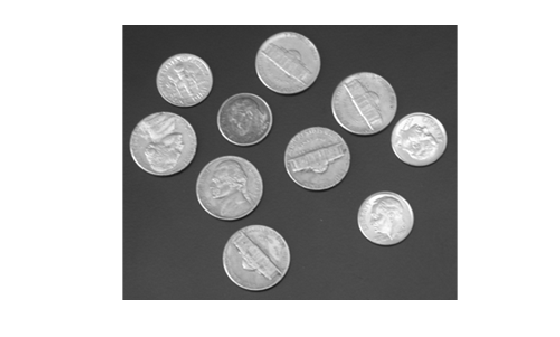## Input Arguments

collapse all

Input image, specified as a numeric array of any dimension.

Example: `I = imread('glass.png');`

Data Types: `single` | `double` | `int8` | `int16` | `int32` | `int64` | `uint8` | `uint16` | `uint32` | `uint64`

H-maxima transform, specified as a nonnegative scalar.

Example: `BW = imextendedmax(I,80);`

Data Types: `single` | `double` | `int8` | `int16` | `int32` | `int64` | `uint8` | `uint16` | `uint32` | `uint64`

Pixel connectivity, specified as one of the values in this table. The default connectivity is `8` for 2-D images, and `26` for 3-D images.

Value

Meaning

Two-Dimensional Connectivities

`4`

Pixels are connected if their edges touch. The neighborhood of a pixel are the adjacent pixels in the horizontal or vertical direction.Current pixel is shown in gray.

`8`

Pixels are connected if their edges or corners touch. The neighborhood of a pixel are the adjacent pixels in the horizontal, vertical, or diagonal direction.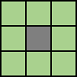Current pixel is shown in gray.

Three-Dimensional Connectivities

`6`

Pixels are connected if their faces touch. The neighborhood of a pixel are the adjacent pixels in:

• One of these directions: in, out, left, right, up, and down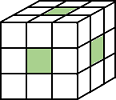Current pixel is shown in gray.

`18`

Pixels are connected if their faces or edges touch. The neighborhood of a pixel are the adjacent pixels in:

• One of these directions: in, out, left, right, up, and down

• A combination of two directions, such as right-down or in-up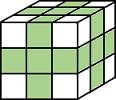Current pixel is center of cube.

`26`

Pixels are connected if their faces, edges, or corners touch. The neighborhood of a pixel are the adjacent pixels in:

• One of these directions: in, out, left, right, up, and down

• A combination of two directions, such as right-down or in-up

• A combination of three directions, such as in-right-up or in-left-down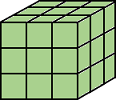Current pixel is center of cube.

For higher dimensions, `imextendedmax` uses the default value `conndef(ndims(I),'maximal')`.

Connectivity can also be defined in a more general way for any dimension by specifying a 3-by-3-by- ... -by-3 matrix of `0`s and `1`s. The `1`-valued elements define neighborhood locations relative to the center element of `conn`. Note that `conn` must be symmetric about its center element. See Specifying Custom Connectivities for more information.

Data Types: `single` | `double` | `int8` | `int16` | `int32` | `int64` | `uint8` | `uint16` | `uint32` | `uint64`

## Output Arguments

collapse all

Transformed image, returned as a logical array the same size as `I`.

 Soille, P. Morphological Image Analysis: Principles and Applications. Springer-Verlag, 1999, pp. 170-171.